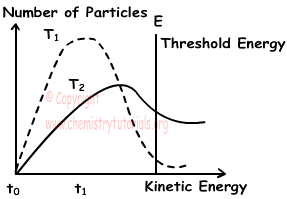## Rates of Reaction Exam1 and Problem Solutions

Rates of Reaction Exam1 and Problem Solutions

1. Which ones of the followings increases rate of reaction in gas phase;

II. Decreasing pressure

III. Increasing temperature

IV. Increasing volume

Solution:

Rate of chemical reaction in gas phase increases with; adding catalysts, increasing pressure or decreasing volume, increasing temperature. Thus, decreasing pressure and increasing volume decreases rate of reaction rate. I and III increases reaction rate.

2. X gas in a container gives following reaction;

2X(g) ↔ Y(g)

Kinetic energies of X gas at temperatures 1 and 2 are given below.If temperature is changed from T1 to T2, which ones of the followings increase?

I. Rate of reaction

II. Number of particles that are activated

III. Average kinetic energySolution:

When you examine the graph, you can see that number of particles at T2 is larger than number of particles at T1. We brush the area that shows number of particles in both temperatures in given graph below;I, II and III are true.

3. Steps of a reaction are given below;

2A + B → 2C  ∆H1 < 0

C + D → A + 2E  ∆H2 < 0

Which ones of the followings are true for this reaction;

I. Reaction is; B + 2D → 4E

II. It is exothermic

III. A and C are catalysts

Solution:

We multiply second reaction with 2 and sum with reaction I.

2A + B → 2C  ∆H1 < 0

2C + 2D → 2A + 4E  ∆H2 < 0

———————————————————————

B + 2D → 4E ∆H < 0

I is true, since ∆H < 0, reaction is exothermic and II is also true. A joins the reaction and leave without any change in its structure, so it is catalyst but C is not catalyst. III is false.

4. Reaction; 2AB2(g) + C2(g) → 2AB2C(g) takes place in two steps. If fast step of this reaction is;

AB2(g) + C(g) → AB2C(g)

find rate of this reaction.

Solution:

If we reverse the fast step and sum it with following reaction we can find slow step of this reaction.

2AB2(g) + C2(g) → 2AB2C(g)

AB2C(g) → AB2(g) + C(g)

———————————————————

AB2(g) + C2(g) → AB2C(g) + C(g) this is slow step of reaction.

Reaction Rate=k.[AB2].[C2]

5. A reaction have slow and fast steps as given below;

I. NO(g) + Cl2(g)  ↔ NOCl2(g) Fast

II. NOCl2(g) + NO(g) → 2NOCl(g)

Which ones of the following statements are true for this reaction?

I. Reaction is; 2NO(g) + Cl2(g) → 2NOCl(g)

II. Reaction Rate = k.[Cl2].[NO]

III. Activation energy of fast step is lower than slow step

Solution:

NO(g) + Cl2(g)  ↔ NOCl2(g) Fast

NOCl2(g) + NO(g) → 2NOCl(g) Slow

———————————————————

Reaction: 2NO(g) + Cl2(g) → 2NOCl(g) I is true.

II. Reaction rate is found using slow step of reaction.

Reaction Rate = k.[NOCl2].[NO] II is false

III. Increasing activation energy decreases reaction rate. III is true.

Related